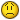# help needed in excel 07

#### Kislay

Any one help me solve this question in excel 2007The Salesworksheet contains 48 months of sales at a toy store. Create an array formula
to add (beginning with Month 3) every fifth month of sales. (Hint: You might want
to use the Excel MOD function. MOD(number,divisor)yields the remainder after the
number is divided by the divisor. For example, MOD(7,5)yields 2. Use concept of Array formulae and function